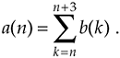# SIGNAL PROCESSING OPERATIONAL SYMBOLS

We'll be using block diagrams to graphically depict the way digital signal-processing operations are implemented. Those block diagrams will comprise an assortment of fundamental processing symbols, the most common of which are illustrated and mathematically defined in Figure 1-6.

Figure 1-6. Terminology and symbols used in digital signal processing block diagrams.Figure 1-6(a) shows the addition, element for element, of two discrete sequences to provide a new sequence. If our sequence index n begins at 0, we say that the first output sequence value is equal to the sum of the first element of the b sequence and the first element of the c sequence, or a(0) = b(0) + c(0). Likewise, the second output sequence value is equal to the sum of the second element of the b sequence and the second element of the c sequence, or a(1) = b(1) + c(1). Equation (1-7) is an example of adding two sequences. The subtraction process in Figure 1-6(b) generates an output sequence that's the element-for-element difference of the two input sequences. There are times when we must calculate a sequence whose elements are the sum of more than two values. This operation, illustrated in Figure 1-6(c), is called summation and is very common in digital signal processing. Notice how the lower and upper limits of the summation index k in the expression in Figure 1-6(c) tell us exactly which elements of the b sequence to sum to obtain a given a(n) value. Because we'll encounter summation operations so often, let's make sure we understand their notation. If we repeat the summation equation from Figure 1-6(c) here we haveThis means that

Equation 1-10We'll begin using summation operations in earnest when we discuss digital filters in Chapter 5.

The multiplication of two sequences is symbolized in Figure 1-6(d). Multiplication generates an output sequence that's the element-for-element product of two input sequences: a(0) = b(0)c(0), a(1) = b(1)c(1), and so on. The last fundamental operation that we'll be using is called the unit delay in Figure 1-6(e). While we don't need to appreciate its importance at this point, we'll merely state that the unit delay symbol signifies an operation where the output sequence a(n) is equal to a delayed version of the b(n) sequence. For example, a(5) = b(4), a(6) = b(5), a(7) = b(6), etc. As we'll see in Chapter 6, due to the mathematical techniques used to analyze digital filters, the unit delay is very often depicted using the term z–1.

The symbols in Figure 1-6 remind us of two important aspects of digital signal processing. First, our processing operations are always performed on sequences of individual discrete values, and second, the elementary operations themselves are very simple. It's interesting that, regardless of how complicated they appear to be, the vast majority of digital signal processing algorithms can be performed using combinations of these simple operations. If we think of a digital signal processing algorithm as a recipe, then the symbols in Figure 1-6 are the ingredients.

URL http://proquest.safaribooksonline.com/0131089897/ch01lev1sec3Amazon